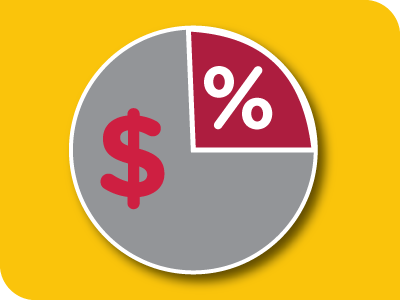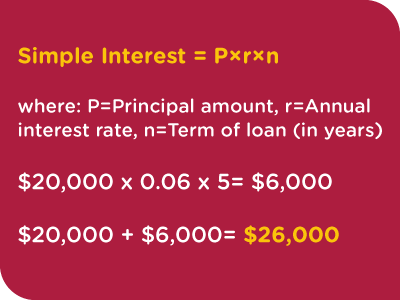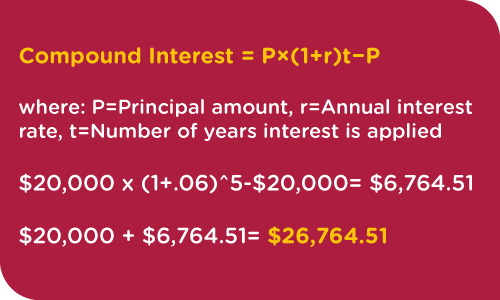# InterestInterest is the fee that you pay to the lender for taking out a loan. There are two types of interest: simple and compound.

Simple Interest is just what the name implies. It’s a simple percentage of the principle amount.

For example, you take out a car loan for \$20,000. Your loan agreement states that you will pay 6% interest and the loan term is for 5 years. You would pay \$6,000 in interest over the lifetime of the loan.The total amount paid for your car is \$26,000.

Compound Interest is a more complicated formula. The interest is calculated or re-calculated a various points in time. The first time the interest is calculated, it is based off of just the principle amount. However, each time interest is re-calculated afterward, the percentage is applied to the remaining principle AND any interest previously accrued. Increments can be recalculated monthly, bi-annually, annually, or a custom period of time.

For example, you take out a car loan for \$20,000. Your loan agreement states that you will pay 6% interest and the loan term is for 5 years. You would pay \$6,764.51 in interest over the lifetime of the loan.The total amount paid for your car loan is \$26,764.51.

Related: Simple vs. Compound Interest: What’s the Difference? (Indeed.com)

Related: What is Compound Interest (NerdWallet.com)

When earning interest, compound interest will result in higher returns than simple interest. However, that works the opposite when dealing with a debt. Compounded interest will add up to more money owed over time than simple interest.

Call 800.492.7120 TO GET STARTED TODAY!